【机器学习】《机器学习实战》读书笔记及代码 总目录

——————————————————————————————————————————————————————

## 本章内容

• 组合相似的分类器来提高分类性能
• 处理非均衡分类问题

### 1、基于数据集多重抽样的分类器 - 集成方法

#### 1）bagging：基于数据随机重抽样的分类器构建方法

Bagging
1.通过降低基分类器的方差，改善了泛化误差。
2.性能依赖于基分类器的稳定性，如果基分类器不稳定，bagging有助于降低训练数据的随机波动导致的误差；如果稳定，则集成分类器的误差主要由基分类器的偏倚引起。
3.由于每个样本被选中的概率相同，因此bagging并不侧重于训练数据集中的任何特定实例。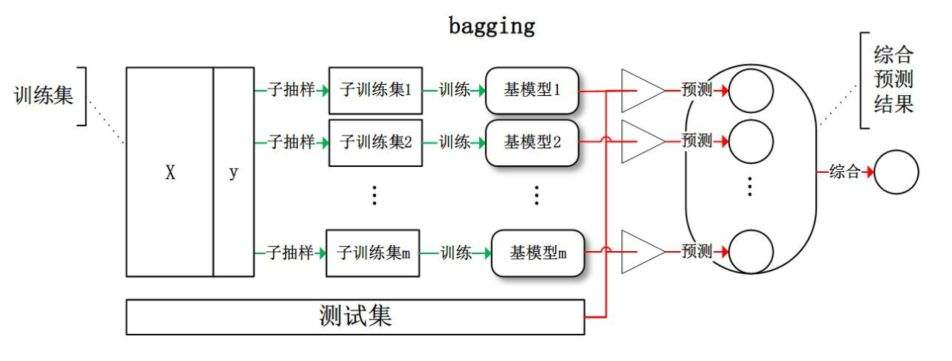#### 2）boosting

boosting是一种与bagging很类似的技术。不论是在boosting还是bagging当中，所使用的多个分类器的类型都是一致的。但是在前者当中，不同的分类器是通过串行训练而获得的，每个新分类器都根据已训练出的分类器的性能来进行训练。

boosting是通过集中关注被已有分类器错分的那些数据来获得新的分类器。由于boosting分类的结果是基于所有分类器的加权求和结果的，因此boosting与bagging不太一样。bagging中的分类器权重是相等的，而boosting中的分类器权重并不相等，每个权重代表的是其对应分类器在上一轮迭代中的成功度#### 3）二者的区别

• 样本选择上：

• Bagging：训练集是在原始集中有放回选取的，从原始集中选出的各轮训练集之间是独立的。
• Boosting：每一轮的训练集不变，只是训练集中每个样例在分类器中的权重发生变化。而权值是根据上一轮的分类结果进行调整。
• 样例权重：

• Bagging：使用均匀取样，每个样例的权重相等。
• Boosting：根据错误率不断调整样例的权值，错误率越大则权重越大。
• 预测函数：

• Bagging：所有预测函数的权重相等。
• Boosting：每个弱分类器都有相应的权重，对于分类误差小的分类器会有更大的权重。
• 并行计算：

• Bagging：各个预测函数可以并行生成。
• Boosting：各个预测函数只能顺序生成，因为后一个模型参数需要前一轮模型的结果。

### 2、提升分类器性能利器 - AdaBoost

(1) 收集数据：可以使用任意方法。
(2) 准备数据：依赖于所使用的弱分类器类型，本章使用的是单层决策树，这种分类器可以处理任何数据类型。当然也可以使用任意分类器作为弱分类器，第2章到第6章中的任一分类器都可以充当弱分类器。作为弱分类器，简单分类器的效果更好。
(3) 分析数据：可以使用任意方法。
(5) 测试算法：计算分类的错误率。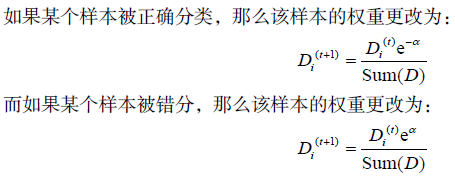### 3、基于单层决策树构建弱分类器

#### 1）数据集可视化

import numpy as np
import matplotlib.pyplot as plt

# 创建单层决策树的数据集
"""
Parameters:
无
Returns:
dataMat - 数据矩阵
classLabels - 数据标签
"""
datMat = np.matrix([[ 1. ,  2.1],
[ 1.5,  1.6],
[ 1.3,  1. ],
[ 1. ,  1. ],
[ 2. ,  1. ]])
classLabels = [1.0, 1.0, -1.0, -1.0, 1.0]
return datMat,classLabels

# 数据可视化
def showDataSet(dataMat, labelMat):
"""
Parameters:
dataMat - 数据矩阵
labelMat - 数据标签
Returns:
无
"""
data_plus = []                                  							#正样本
data_minus = []                                 							#负样本
for i in range(len(dataMat)):
if labelMat[i] > 0:
data_plus.append(dataMat[i])
else:
data_minus.append(dataMat[i])
data_plus_np = np.array(data_plus)                                          #转换为numpy矩阵
data_minus_np = np.array(data_minus)                                        #转换为numpy矩阵
plt.scatter(np.transpose(data_plus_np), np.transpose(data_plus_np))   #正样本散点图
plt.scatter(np.transpose(data_minus_np), np.transpose(data_minus_np)) #负样本散点图
plt.show()

if __name__ == '__main__':
showDataSet(dataArr,classLabels)#### 2）构建单层决策树

import numpy as np
import matplotlib.pyplot as plt

# 创建单层决策树的数据集
"""
Parameters:
无
Returns:
dataMat - 数据矩阵
classLabels - 数据标签
"""
datMat = np.matrix([[ 1. ,  2.1],
[ 1.5,  1.6],
[ 1.3,  1. ],
[ 1. ,  1. ],
[ 2. ,  1. ]])
classLabels = [1.0, 1.0, -1.0, -1.0, 1.0]
return datMat,classLabels

# 单层决策树分类函数
def stumpClassify(dataMatrix,dimen,threshVal,threshIneq):
"""
Parameters:
dataMatrix - 数据矩阵
dimen - 第dimen列，也就是第几个特征
threshVal - 阈值
threshIneq - 标志
Returns:
retArray - 分类结果
"""
retArray = np.ones((np.shape(dataMatrix),1))         #初始化retArray为1
if threshIneq == 'lt':
retArray[dataMatrix[:,dimen] <= threshVal] = -1.0   #如果小于阈值,则赋值为-1
else:
retArray[dataMatrix[:,dimen] > threshVal] = -1.0    #如果大于阈值,则赋值为-1
return retArray

# 找到数据集上最佳的单层决策树
def buildStump(dataArr,classLabels,D):
"""
Parameters:
dataArr - 数据矩阵
classLabels - 数据标签
D - 样本权重
Returns:
bestStump - 最佳单层决策树信息
minError - 最小误差
bestClasEst - 最佳的分类结果
"""
dataMatrix = np.mat(dataArr); labelMat = np.mat(classLabels).T
m,n = np.shape(dataMatrix)
numSteps = 10.0; bestStump = {}; bestClasEst = np.mat(np.zeros((m,1)))
minError = float('inf')                                                     #最小误差初始化为正无穷大
for i in range(n):                                                          #遍历所有特征
rangeMin = dataMatrix[:,i].min(); rangeMax = dataMatrix[:,i].max()      #找到特征中最小的值和最大值
stepSize = (rangeMax - rangeMin) / numSteps                             #计算步长
for j in range(-1, int(numSteps) + 1):
for inequal in ['lt', 'gt']:                                        #大于和小于的情况，均遍历。lt:less than，gt:greater than
threshVal = (rangeMin + float(j) * stepSize)                    #计算阈值
predictedVals = stumpClassify(dataMatrix, i, threshVal, inequal)#计算分类结果
errArr = np.mat(np.ones((m,1)))                                 #初始化误差矩阵
errArr[predictedVals == labelMat] = 0                           #分类正确的,赋值为0
weightedError = D.T * errArr                                    #计算误差
print("split: dim %d, thresh %.2f, thresh ineqal: %s, the weighted error is %.3f" % (i, threshVal, inequal, weightedError))
if weightedError < minError:                                    #找到误差最小的分类方式
minError = weightedError
bestClasEst = predictedVals.copy()
bestStump['dim'] = i
bestStump['thresh'] = threshVal
bestStump['ineq'] = inequal
return bestStump,minError,bestClasEst

if __name__ == '__main__':
D = np.mat(np.ones((5, 1)) / 5)
bestStump,minError,bestClasEst = buildStump(dataArr,classLabels,D)
print('bestStump:\n', bestStump)
print('minError:\n', minError)
print('bestClasEst:\n', bestClasEst)对每次迭代：
利用buildStump()函数找到最佳的单层决策树
将最佳单层决策树加入到单层决策树数组
计算alpha
计算新的权重向量D
更新累计类别估计值
如果错误率等于0.0，则退出循环


import numpy as np
import matplotlib.pyplot as plt

# 创建单层决策树的数据集
"""
Parameters:
无
Returns:
dataMat - 数据矩阵
classLabels - 数据标签
"""
datMat = np.matrix([[ 1. ,  2.1],
[ 1.5,  1.6],
[ 1.3,  1. ],
[ 1. ,  1. ],
[ 2. ,  1. ]])
classLabels = [1.0, 1.0, -1.0, -1.0, 1.0]
return datMat,classLabels

# 单层决策树分类函数
def stumpClassify(dataMatrix,dimen,threshVal,threshIneq):
"""
Parameters:
dataMatrix - 数据矩阵
dimen - 第dimen列，也就是第几个特征
threshVal - 阈值
threshIneq - 标志
Returns:
retArray - 分类结果
"""
retArray = np.ones((np.shape(dataMatrix),1))       #初始化retArray为1
if threshIneq == 'lt':
retArray[dataMatrix[:,dimen] <= threshVal] = -1.0 #如果小于阈值,则赋值为-1
else:
retArray[dataMatrix[:,dimen] > threshVal] = -1.0  #如果大于阈值,则赋值为-1
return retArray

# 找到数据集上最佳的单层决策树
def buildStump(dataArr,classLabels,D):
"""
Parameters:
dataArr - 数据矩阵
classLabels - 数据标签
D - 样本权重
Returns:
bestStump - 最佳单层决策树信息
minError - 最小误差
bestClasEst - 最佳的分类结果
"""
dataMatrix = np.mat(dataArr); labelMat = np.mat(classLabels).T
m,n = np.shape(dataMatrix)
numSteps = 10.0; bestStump = {}; bestClasEst = np.mat(np.zeros((m,1)))
minError = float('inf')                                                      #最小误差初始化为正无穷大
for i in range(n):                                                           #遍历所有特征
rangeMin = dataMatrix[:,i].min(); rangeMax = dataMatrix[:,i].max()       #找到特征中最小的值和最大值
stepSize = (rangeMax - rangeMin) / numSteps                              #计算步长
for j in range(-1, int(numSteps) + 1):
for inequal in ['lt', 'gt']:                                         #大于和小于的情况，均遍历。lt:less than，gt:greater than
threshVal = (rangeMin + float(j) * stepSize)                     #计算阈值
predictedVals = stumpClassify(dataMatrix, i, threshVal, inequal) #计算分类结果
errArr = np.mat(np.ones((m,1)))                                  #初始化误差矩阵
errArr[predictedVals == labelMat] = 0                            #分类正确的,赋值为0
weightedError = D.T * errArr                                     #计算误差
print("split: dim %d, thresh %.2f, thresh ineqal: %s, the weighted error is %.3f" % (i, threshVal, inequal, weightedError))
if weightedError < minError:                                     #找到误差最小的分类方式
minError = weightedError
bestClasEst = predictedVals.copy()
bestStump['dim'] = i
bestStump['thresh'] = threshVal
bestStump['ineq'] = inequal
return bestStump, minError, bestClasEst

def adaBoostTrainDS(dataArr, classLabels, numIt = 40):
weakClassArr = []
m = np.shape(dataArr)
D = np.mat(np.ones((m, 1)) / m)                                       #初始化权重
aggClassEst = np.mat(np.zeros((m,1)))
for i in range(numIt):
bestStump, error, classEst = buildStump(dataArr, classLabels, D)  #构建单层决策树
print("D:",D.T)
alpha = float(0.5 * np.log((1.0 - error) / max(error, 1e-16)))    #计算弱学习算法权重alpha,使error不等于0,因为分母不能为0
bestStump['alpha'] = alpha                                        #存储弱学习算法权重
weakClassArr.append(bestStump)                                    #存储单层决策树
print("classEst: ", classEst.T)
expon = np.multiply(-1 * alpha * np.mat(classLabels).T, classEst) #计算e的指数项
D = np.multiply(D, np.exp(expon))
D = D / D.sum()                                                   #根据样本权重公式，更新样本权重
aggClassEst += alpha * classEst
print("aggClassEst: ", aggClassEst.T)
aggErrors = np.multiply(np.sign(aggClassEst) != np.mat(classLabels).T, np.ones((m,1)))#计算误差
errorRate = aggErrors.sum() / m
print("total error: ", errorRate)
if errorRate == 0.0: break                                        #误差为0，退出循环
return weakClassArr, aggClassEst

if __name__ == '__main__':
weakClassArr, aggClassEst = adaBoostTrainDS(dataArr, classLabels)
print(weakClassArr)
print(aggClassEst)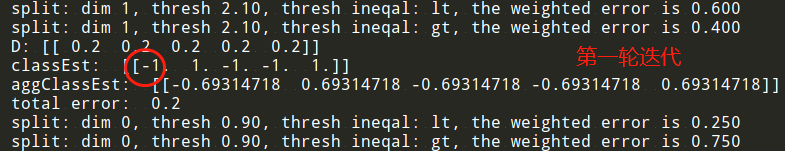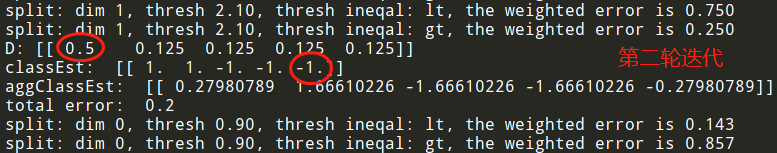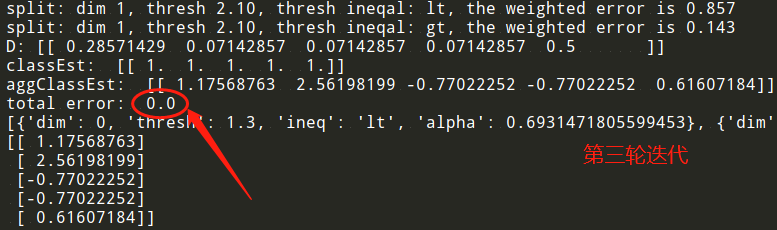import numpy as np
import matplotlib.pyplot as plt

# 创建单层决策树的数据集
"""
Parameters:
无
Returns:
dataMat - 数据矩阵
classLabels - 数据标签
"""
datMat = np.matrix([[ 1. ,  2.1],
[ 1.5,  1.6],
[ 1.3,  1. ],
[ 1. ,  1. ],
[ 2. ,  1. ]])
classLabels = [1.0, 1.0, -1.0, -1.0, 1.0]
return datMat,classLabels

# 数据可视化
def showDataSet(dataMat, labelMat):
"""
Parameters:
dataMat - 数据矩阵
labelMat - 数据标签
Returns:
无
"""
data_plus = []                                  							#正样本
data_minus = []                                 							#负样本
for i in range(len(dataMat)):
if labelMat[i] > 0:
data_plus.append(dataMat[i])
else:
data_minus.append(dataMat[i])
data_plus_np = np.array(data_plus)                                          #转换为numpy矩阵
data_minus_np = np.array(data_minus)                                        #转换为numpy矩阵
plt.scatter(np.transpose(data_plus_np), np.transpose(data_plus_np))   #正样本散点图
plt.scatter(np.transpose(data_minus_np), np.transpose(data_minus_np)) #负样本散点图
plt.show()

# 单层决策树分类函数
def stumpClassify(dataMatrix,dimen,threshVal,threshIneq):
"""
Parameters:
dataMatrix - 数据矩阵
dimen - 第dimen列，也就是第几个特征
threshVal - 阈值
threshIneq - 标志
Returns:
retArray - 分类结果
"""
retArray = np.ones((np.shape(dataMatrix),1))        #初始化retArray为1
if threshIneq == 'lt':
retArray[dataMatrix[:,dimen] <= threshVal] = -1.0  #如果小于阈值,则赋值为-1
else:
retArray[dataMatrix[:,dimen] > threshVal] = -1.0   #如果大于阈值,则赋值为-1
return retArray

# 找到数据集上最佳的单层决策树
def buildStump(dataArr,classLabels,D):
"""
Parameters:
dataArr - 数据矩阵
classLabels - 数据标签
D - 样本权重
Returns:
bestStump - 最佳单层决策树信息
minError - 最小误差
bestClasEst - 最佳的分类结果
"""
dataMatrix = np.mat(dataArr); labelMat = np.mat(classLabels).T
m,n = np.shape(dataMatrix)
numSteps = 10.0; bestStump = {}; bestClasEst = np.mat(np.zeros((m,1)))
minError = float('inf')                                                     #最小误差初始化为正无穷大
for i in range(n):                                                          #遍历所有特征
rangeMin = dataMatrix[:,i].min(); rangeMax = dataMatrix[:,i].max()      #找到特征中最小的值和最大值
stepSize = (rangeMax - rangeMin) / numSteps                             #计算步长
for j in range(-1, int(numSteps) + 1):
for inequal in ['lt', 'gt']:                                        #大于和小于的情况，均遍历。lt:less than，gt:greater than
threshVal = (rangeMin + float(j) * stepSize)                    #计算阈值
predictedVals = stumpClassify(dataMatrix, i, threshVal, inequal)#计算分类结果
errArr = np.mat(np.ones((m,1)))                                 #初始化误差矩阵
errArr[predictedVals == labelMat] = 0                           #分类正确的,赋值为0
weightedError = D.T * errArr                                    #计算误差
# print("split: dim %d, thresh %.2f, thresh ineqal: %s, the weighted error is %.3f" % (i, threshVal, inequal, weightedError))
if weightedError < minError:                                   #找到误差最小的分类方式
minError = weightedError
bestClasEst = predictedVals.copy()
bestStump['dim'] = i
bestStump['thresh'] = threshVal
bestStump['ineq'] = inequal
return bestStump, minError, bestClasEst

def adaBoostTrainDS(dataArr, classLabels, numIt = 40):
"""
Parameters:
dataArr - 数据矩阵
classLabels - 数据标签
numIt - 最大迭代次数
Returns:
weakClassArr - 训练好的分类器
aggClassEst - 类别估计累计值
"""
weakClassArr = []
m = np.shape(dataArr)
D = np.mat(np.ones((m, 1)) / m)                                       #初始化权重
aggClassEst = np.mat(np.zeros((m,1)))
for i in range(numIt):
bestStump, error, classEst = buildStump(dataArr, classLabels, D)  #构建单层决策树
# print("D:",D.T)
alpha = float(0.5 * np.log((1.0 - error) / max(error, 1e-16)))    #计算弱学习算法权重alpha,使error不等于0,因为分母不能为0
bestStump['alpha'] = alpha                                        #存储弱学习算法权重
weakClassArr.append(bestStump)                                    #存储单层决策树
# print("classEst: ", classEst.T)
expon = np.multiply(-1 * alpha * np.mat(classLabels).T, classEst) #计算e的指数项
D = np.multiply(D, np.exp(expon))
D = D / D.sum()                                                   #根据样本权重公式，更新样本权重
aggClassEst += alpha * classEst                                   #计算类别估计累计值
# print("aggClassEst: ", aggClassEst.T)
aggErrors = np.multiply(np.sign(aggClassEst) != np.mat(classLabels).T, np.ones((m,1))) #计算误差
errorRate = aggErrors.sum() / m
# print("total error: ", errorRate)
if errorRate == 0.0: break                                        #误差为0，退出循环
return weakClassArr, aggClassEst

"""
Parameters:
datToClass - 待分类样例
classifierArr - 训练好的分类器
Returns:
分类结果
"""
dataMatrix = np.mat(datToClass)
m = np.shape(dataMatrix)
aggClassEst = np.mat(np.zeros((m,1)))
for i in range(len(classifierArr)):                                        #遍历所有分类器，进行分类
classEst = stumpClassify(dataMatrix, classifierArr[i]['dim'], classifierArr[i]['thresh'], classifierArr[i]['ineq'])
aggClassEst += classifierArr[i]['alpha'] * classEst
print(aggClassEst)
return np.sign(aggClassEst)

if __name__ == '__main__':
weakClassArr, aggClassEst = adaBoostTrainDS(dataArr, classLabels)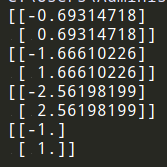(1) 收集数据：提供的文本文件。
(2) 准备数据：确保类别标签是+1和1而非1和0。
(3) 分析数据：手工检查数据。
(6) 使用算法：观察该例子上的错误率。不过，也可以构建一个Web网站，让驯马师输入马的症状然后预测马是否会死去。

《机器学习实战》学习笔记（五）：Logistic 回归中，我们使用了Logistic回归方法训练马疝病数据集，预测病马死亡率。当时的训练结果如下图所示：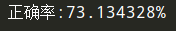import numpy as np
import matplotlib.pyplot as plt

dataMat = []; labelMat = []
fr = open(fileName)
for line in fr.readlines():
lineArr = []
curLine = line.strip().split('\t')
for i in range(numFeat - 1):
lineArr.append(float(curLine[i]))
dataMat.append(lineArr)
labelMat.append(float(curLine[-1]))
return dataMat, labelMat

# 单层决策树分类函数
def stumpClassify(dataMatrix,dimen,threshVal,threshIneq):
"""
Parameters:
dataMatrix - 数据矩阵
dimen - 第dimen列，也就是第几个特征
threshVal - 阈值
threshIneq - 标志
Returns:
retArray - 分类结果
"""
retArray = np.ones((np.shape(dataMatrix),1))       #初始化retArray为1
if threshIneq == 'lt':
retArray[dataMatrix[:,dimen] <= threshVal] = -1.0 #如果小于阈值,则赋值为-1
else:
retArray[dataMatrix[:,dimen] > threshVal] = -1.0  #如果大于阈值,则赋值为-1
return retArray

# 找到数据集上最佳的单层决策树
def buildStump(dataArr,classLabels,D):
"""
Parameters:
dataArr - 数据矩阵
classLabels - 数据标签
D - 样本权重
Returns:
bestStump - 最佳单层决策树信息
minError - 最小误差
bestClasEst - 最佳的分类结果
"""
dataMatrix = np.mat(dataArr); labelMat = np.mat(classLabels).T
m,n = np.shape(dataMatrix)
numSteps = 10.0; bestStump = {}; bestClasEst = np.mat(np.zeros((m,1)))
minError = float('inf')                                                     #最小误差初始化为正无穷大
for i in range(n):                                                          #遍历所有特征
rangeMin = dataMatrix[:,i].min(); rangeMax = dataMatrix[:,i].max()      #找到特征中最小的值和最大值
stepSize = (rangeMax - rangeMin) / numSteps                             #计算步长
for j in range(-1, int(numSteps) + 1):
for inequal in ['lt', 'gt']:                                        #大于和小于的情况，均遍历。lt:less than，gt:greater than
threshVal = (rangeMin + float(j) * stepSize)                    #计算阈值
predictedVals = stumpClassify(dataMatrix, i, threshVal, inequal)#计算分类结果
errArr = np.mat(np.ones((m,1)))                                 #初始化误差矩阵
errArr[predictedVals == labelMat] = 0                           #分类正确的,赋值为0
weightedError = D.T * errArr                                    #计算误差
# print("split: dim %d, thresh %.2f, thresh ineqal: %s, the weighted error is %.3f" % (i, threshVal, inequal, weightedError))
if weightedError < minError:                                    #找到误差最小的分类方式
minError = weightedError
bestClasEst = predictedVals.copy()
bestStump['dim'] = i
bestStump['thresh'] = threshVal
bestStump['ineq'] = inequal
return bestStump, minError, bestClasEst

def adaBoostTrainDS(dataArr, classLabels, numIt = 40):
"""
Parameters:
dataArr - 数据矩阵
classLabels - 数据标签
numIt - 最大迭代次数
Returns:
weakClassArr - 训练好的分类器
aggClassEst - 类别估计累计值
"""
weakClassArr = []
m = np.shape(dataArr)
D = np.mat(np.ones((m, 1)) / m)                                       #初始化权重
aggClassEst = np.mat(np.zeros((m,1)))
for i in range(numIt):
bestStump, error, classEst = buildStump(dataArr, classLabels, D)  #构建单层决策树
# print("D:",D.T)
alpha = float(0.5 * np.log((1.0 - error) / max(error, 1e-16)))    #计算弱学习算法权重alpha,使error不等于0,因为分母不能为0
bestStump['alpha'] = alpha                                        #存储弱学习算法权重
weakClassArr.append(bestStump)                                    #存储单层决策树
# print("classEst: ", classEst.T)
expon = np.multiply(-1 * alpha * np.mat(classLabels).T, classEst) #计算e的指数项
D = np.multiply(D, np.exp(expon))
D = D / D.sum()                                                   #根据样本权重公式，更新样本权重
aggClassEst += alpha * classEst                                   #计算类别估计累计值
# print("aggClassEst: ", aggClassEst.T)
aggErrors = np.multiply(np.sign(aggClassEst) != np.mat(classLabels).T, np.ones((m,1)))#计算误差
errorRate = aggErrors.sum() / m
# print("total error: ", errorRate)
if errorRate == 0.0: break                                        #误差为0，退出循环
return weakClassArr, aggClassEst

"""
Parameters:
datToClass - 待分类样例
classifierArr - 训练好的分类器
Returns:
分类结果
"""
dataMatrix = np.mat(datToClass)
m = np.shape(dataMatrix)
aggClassEst = np.mat(np.zeros((m,1)))
print(len(classifierArr))
for i in range(len(classifierArr)):                                   #遍历所有分类器，进行分类
classEst = stumpClassify(dataMatrix, classifierArr[i]['dim'], classifierArr[i]['thresh'], classifierArr[i]['ineq'])
aggClassEst += classifierArr[i]['alpha'] * classEst
# print(aggClassEst)
return np.sign(aggClassEst)

if __name__ == '__main__':
dataArr, LabelArr = loadDataSet('horseColicTraining2.txt')
weakClassArr, aggClassEst = adaBoostTrainDS(dataArr, LabelArr)
testArr, testLabelArr = loadDataSet('horseColicTest2.txt')
print(weakClassArr)
predictions = adaClassify(dataArr, weakClassArr)
errArr = np.mat(np.ones((len(dataArr), 1)))
print('训练集的错误率:%.3f%%' % float(errArr[predictions != np.mat(LabelArr).T].sum() / len(dataArr) * 100))
predictions = adaClassify(testArr, weakClassArr)
errArr = np.mat(np.ones((len(testArr), 1)))
print('测试集的错误率:%.3f%%' % float(errArr[predictions != np.mat(testLabelArr).T].sum() / len(testArr) * 100))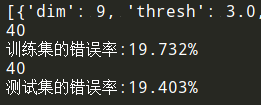import numpy as np
from sklearn.ensemble import AdaBoostClassifier
from sklearn.tree import DecisionTreeClassifier

dataMat = []; labelMat = []
fr = open(fileName)
for line in fr.readlines():
lineArr = []
curLine = line.strip().split('\t')
for i in range(numFeat - 1):
lineArr.append(float(curLine[i]))
dataMat.append(lineArr)
labelMat.append(float(curLine[-1]))
return dataMat, labelMat

if __name__ == '__main__':
dataArr, classLabels = loadDataSet('horseColicTraining2.txt')
testArr, testLabelArr = loadDataSet('horseColicTest2.txt')
bdt = AdaBoostClassifier(DecisionTreeClassifier(max_depth = 2), algorithm = "SAMME", n_estimators = 10)
bdt.fit(dataArr, classLabels)
predictions = bdt.predict(dataArr)
errArr = np.mat(np.ones((len(dataArr), 1)))
print('训练集的错误率:%.3f%%' % float(errArr[predictions != classLabels].sum() / len(dataArr) * 100))
predictions = bdt.predict(testArr)
errArr = np.mat(np.ones((len(testArr), 1)))
print('测试集的错误率:%.3f%%' % float(errArr[predictions != testLabelArr].sum() / len(testArr) * 100))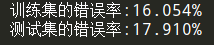### 8、分类器性能评价

• 假如某人给我们牵来一匹马，他希望我们能预测这匹马能否生存。我们说马会死，那么他们就可能会对马实施安乐死，而不是通过给马喂药来延缓其不可避免的死亡过程。我们的预测也许是错误的，马本来是可以继续活着的。毕竟，我们的分类器只有80%的精确率（accuracy）。如果我们预测错误，那么我们将会错杀了一个如此昂贵的动物，更不要说人对马还存在情感上的依恋。
• 如何过滤垃圾邮件呢？如果收件箱中会出现某些垃圾邮件，但合法邮件永远不会扔进垃圾邮件夹中，那么人们是否会满意呢？
• 癌症检测又如何呢？只要患病的人不会得不到治疗，那么再找一个医生来看看会不会更好呢（即情愿误判也不漏判）？

#### 1）分类性能度量指标：正确率、召回率及ROC 曲线ROC曲线不但可以用于比较分类器，还可以基于 成本效益（cost-versus-benefit） 分析来做出决策。由于在不同的阈值下，不同的分类器的表现情况可能各不相同，因此以某种方式将它们组合起来或许会更有意义。如果只是简单地观察分类器的错误率，那么我们就难以得到这种更深入的洞察效果了。

import numpy as np
import matplotlib.pyplot as plt
from matplotlib.font_manager import FontProperties

dataMat = []; labelMat = []
fr = open(fileName)
for line in fr.readlines():
lineArr = []
curLine = line.strip().split('\t')
for i in range(numFeat - 1):
lineArr.append(float(curLine[i]))
dataMat.append(lineArr)
labelMat.append(float(curLine[-1]))

return dataMat, labelMat

# 单层决策树分类函数
def stumpClassify(dataMatrix,dimen,threshVal,threshIneq):
"""
Parameters:
dataMatrix - 数据矩阵
dimen - 第dimen列，也就是第几个特征
threshVal - 阈值
threshIneq - 标志
Returns:
retArray - 分类结果
"""
retArray = np.ones((np.shape(dataMatrix),1))          #初始化retArray为1
if threshIneq == 'lt':
retArray[dataMatrix[:,dimen] <= threshVal] = -1.0    #如果小于阈值,则赋值为-1
else:
retArray[dataMatrix[:,dimen] > threshVal] = -1.0     #如果大于阈值,则赋值为-1
return retArray

# 找到数据集上最佳的单层决策树
def buildStump(dataArr,classLabels,D):
"""
Parameters:
dataArr - 数据矩阵
classLabels - 数据标签
D - 样本权重
Returns:
bestStump - 最佳单层决策树信息
minError - 最小误差
bestClasEst - 最佳的分类结果
"""
dataMatrix = np.mat(dataArr); labelMat = np.mat(classLabels).T
m,n = np.shape(dataMatrix)
numSteps = 10.0; bestStump = {}; bestClasEst = np.mat(np.zeros((m,1)))
minError = float('inf')                                                     #最小误差初始化为正无穷大
for i in range(n):                                                          #遍历所有特征
rangeMin = dataMatrix[:,i].min(); rangeMax = dataMatrix[:,i].max()      #找到特征中最小的值和最大值
stepSize = (rangeMax - rangeMin) / numSteps                             #计算步长
for j in range(-1, int(numSteps) + 1):
for inequal in ['lt', 'gt']:                                        #大于和小于的情况，均遍历。lt:less than，gt:greater than
threshVal = (rangeMin + float(j) * stepSize)                    #计算阈值
predictedVals = stumpClassify(dataMatrix, i, threshVal, inequal)#计算分类结果
errArr = np.mat(np.ones((m,1)))                                 #初始化误差矩阵
errArr[predictedVals == labelMat] = 0                           #分类正确的,赋值为0
weightedError = D.T * errArr                                    #计算误差
# print("split: dim %d, thresh %.2f, thresh ineqal: %s, the weighted error is %.3f" % (i, threshVal, inequal, weightedError))
if weightedError < minError:                                    #找到误差最小的分类方式
minError = weightedError
bestClasEst = predictedVals.copy()
bestStump['dim'] = i
bestStump['thresh'] = threshVal
bestStump['ineq'] = inequal
return bestStump, minError, bestClasEst

def adaBoostTrainDS(dataArr, classLabels, numIt = 40):
"""
Parameters:
dataArr - 数据矩阵
classLabels - 数据标签
numIt - 最大迭代次数
Returns:
weakClassArr - 训练好的分类器
aggClassEst - 类别估计累计值
"""
weakClassArr = []
m = np.shape(dataArr)
D = np.mat(np.ones((m, 1)) / m)                                      #初始化权重
aggClassEst = np.mat(np.zeros((m,1)))
for i in range(numIt):
bestStump, error, classEst = buildStump(dataArr, classLabels, D) #构建单层决策树
# print("D:",D.T)
alpha = float(0.5 * np.log((1.0 - error) / max(error, 1e-16)))   #计算弱学习算法权重alpha,使error不等于0,因为分母不能为0
bestStump['alpha'] = alpha                                       #存储弱学习算法权重
weakClassArr.append(bestStump)                                   #存储单层决策树
# print("classEst: ", classEst.T)
expon = np.multiply(-1 * alpha * np.mat(classLabels).T, classEst)#计算e的指数项
D = np.multiply(D, np.exp(expon))
D = D / D.sum()                                                  #根据样本权重公式，更新样本权重
aggClassEst += alpha * classEst                                  #计算类别估计累计值
# print("aggClassEst: ", aggClassEst.T)
aggErrors = np.multiply(np.sign(aggClassEst) != np.mat(classLabels).T, np.ones((m,1)))#计算误差
errorRate = aggErrors.sum() / m
# print("total error: ", errorRate)
if errorRate == 0.0: break                                       #误差为0，退出循环
return weakClassArr, aggClassEst

# 绘制ROC
def plotROC(predStrengths, classLabels):
"""
Parameters:
predStrengths - 分类器的预测强度
classLabels - 类别
Returns:
无
"""
font = FontProperties(fname=r"c:\windows\fonts\simsun.ttc", size=14)
cur = (1.0, 1.0)                                    #绘制光标的位置
ySum = 0.0                                          #用于计算AUC
numPosClas = np.sum(np.array(classLabels) == 1.0)   #统计正类的数量
yStep = 1 / float(numPosClas)                       #y轴步长
xStep = 1 / float(len(classLabels) - numPosClas)    #x轴步长

sortedIndicies = predStrengths.argsort()            #预测强度排序

fig = plt.figure()
fig.clf()
ax = plt.subplot(111)
for index in sortedIndicies.tolist():
if classLabels[index] == 1.0:
delX = 0; delY = yStep
else:
delX = xStep; delY = 0
ySum += cur                                                #高度累加
ax.plot([cur, cur - delX], [cur, cur - delY], c = 'b')#绘制ROC
cur = (cur - delX, cur - delY)                              #更新绘制光标的位置
ax.plot([0,1], [0,1], 'b--')
plt.title('AdaBoost马疝病检测系统的ROC曲线', FontProperties = font)
plt.xlabel('假阳率', FontProperties = font)
plt.ylabel('真阳率', FontProperties = font)
ax.axis([0, 1, 0, 1])
print('AUC面积为:', ySum * xStep)                                      #计算AUC
plt.show()

if __name__ == '__main__':
dataArr, LabelArr = loadDataSet('horseColicTraining2.txt')
weakClassArr, aggClassEst = adaBoostTrainDS(dataArr, LabelArr)
plotROC(aggClassEst.T, LabelArr)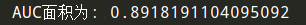#### 2）基于代价函数的分类器决策控制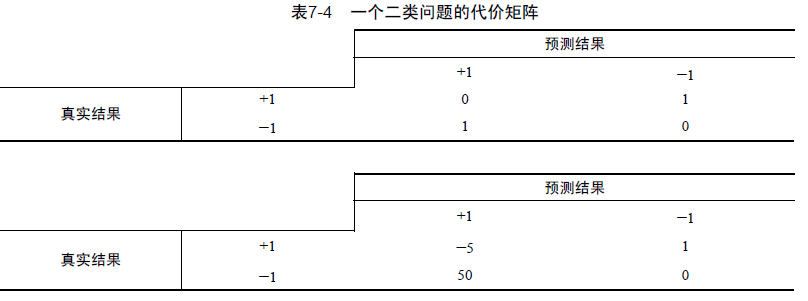### 参考文章